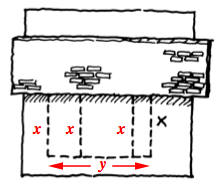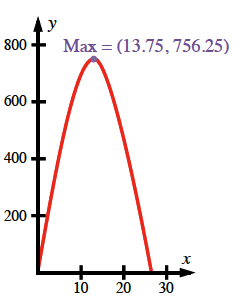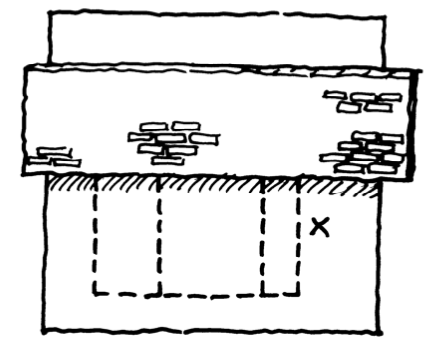### Home > PC > Chapter 9 > Lesson 9.3.3 > Problem9-130

9-130.

You have $110$ meters of fence to enclose and partition the rectangular area against a wall as shown in this top view.

1. Label all sides of the diagram.2. Express the area in terms of $x$.

$4x + y = 110$
$y = 110 −4x$
area $= xy$
area $= x\left(110 − 4x\right)$

3. Graph the area function and use the TRACE button on your calculator to determine the maximum area.